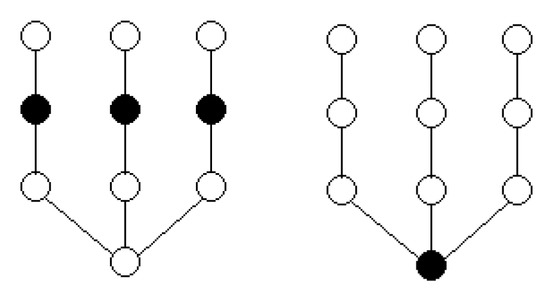Next Article in Journal
A Two-Factor Autoregressive Moving Average Model Based on Fuzzy Fluctuation Logical Relationships
Next Article in Special Issue
Mathematical Properties on the Hyperbolicity of Interval Graphs
Previous Article in Journal
Chiral Separation in Preparative Scale: A Brief Overview of Membranes as Tools for Enantiomeric Separation
Previous Article in Special Issue
Generalized Chordality, Vertex Separators and Hyperbolicity on Graphs
Open AccessArticle

# β-Differential of a Graph

byLudwin A. Basilio 1,Sergio Bermudo 2,Jesús Leaños 1 andJosé M. Sigarreta 3,*
1
Academic Unit of Mathematics, Autonomous University of Zacatecas, Paseo la Bufa, int. Calzada Solidaridad, 98060 Zacatecas, Mexico
2
Department of Economics, Quantitative Methods and Economic History, Pablo de Olavide University, Carretera de Utrera Km. 1, 41013 Sevilla, Spain
3
Faculty of Mathematics, Autonomous University of Guerrero, Carlos E. Adame 5, Col. La Garita, 39350 Acapulco, Guerrero, Mexico
*
Author to whom correspondence should be addressed.
Symmetry 2017, 9(10), 205; https://doi.org/10.3390/sym9100205
Received: 12 September 2017 / Revised: 26 September 2017 / Accepted: 26 September 2017 / Published: 30 September 2017
Let $G = ( V , E )$ be a simple graph with vertex set V and edge set E. Let D be a subset of V, and let $B ( D )$ be the set of neighbours of D in $V ∖ D$ . The differential $∂ ( D )$ of D is defined as $| B ( D ) | − | D |$ . The maximum value of $∂ ( D )$ taken over all subsets $D ⊆ V$ is the differential $∂ ( G )$ of G. For $β ∈ ( − 1 , Δ )$ , the β-differential $∂ β ( G )$ of G is the maximum value of ${ | B ( D ) | − β | D | : D ⊆ V }$ . Motivated by an influential maximization problem, in this paper we study the $β$ -differential of G. View Full-Text
Keywords:
Show FiguresFigure 1

MDPI and ACS Style

Basilio, L.A.; Bermudo, S.; Leaños, J.; Sigarreta, J.M. β-Differential of a Graph. Symmetry 2017, 9, 205.